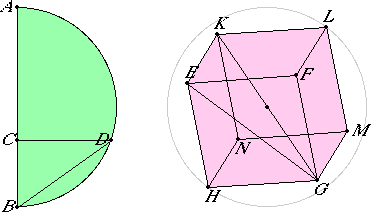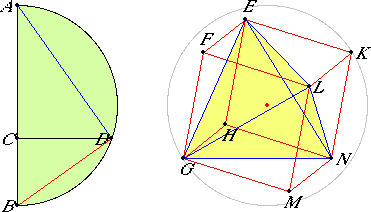# Proposition 15

To construct a cube and comprehend it in a sphere, like the pyramid; and to prove that the square on the diameter of the sphere is triple the square on the side of the cube.

Set out the diameter AB of the given sphere, and cut it at C so that AC is double CB. Describe the semicircle ADB on AB, draw CD from C at right angles to AB, and join DB. Set out the square EFGH having its side equal to DB, draw EK, FL, GM, and HN from E, F, G, and H at right angles to the plane of the square EFGH, and cut EK, FL, GM, and HN off from EK, FL, GM, and HN respectively equal to one of the straight lines EF, FG, GH, or HE. Join KL, LM, MN, and NK.XI.Def.25

Therefore the cube FN has been constructed which is contained by six equal squares.

It is then required to comprehend it in the given sphere, and to prove that the square on the diameter of the sphere is triple the square on the side of the cube.

Join KG and EG.

XI.Def.3

Then, since the angle KEG is right, for KE is also at right angles to the plane EG and of course to the straight line EG also, therefore the semicircle described on KG passes through the point E.

Again, since GF is at right angles to each of the straight lines FL and FE, therefore GF is also at right angles to the plane FK. Hence also, if we join FK, then GF will be at right angles to FK. For this reason the semicircle described on GK also passes through F.

Similarly it also passes through the remaining angular points of the cube.

If then, KG remaining fixed, the semicircle is carried round and restored to the same position from which it began to be moved, then the cube is comprehended in a sphere.

I say next that it is also comprehended in the given sphere.

I.47

For, since GF equals FE, and the angle at F is right, therefore the square on EG is double the square on EF. But EF equals EK, therefore the square on EG is double the square on EK. Hence the sum of the squares on GE and EK, that is the square on GK, is triple the square on EK.

And, since AB is triple BC, while AB is to BC as the square on AB is to the square on BD, therefore the square on AB is triple the square on BD.

But the square on GK was also proved triple the square on KE. And KE was made equal to DB, therefore KG also equals AB. And AB is the diameter of the given sphere, therefore KG also equals the diameter of the given sphere.

Therefore the cube has been comprehended in the given sphere, and it has been demonstrated at the same time that the square on the diameter of the sphere is triple the square on the side of the cube.

Q.E.F.

## Guide

#### Special relationships between regular tetrahedra and cubes

Note that the beginning of this construction of a cube is the same as that for the tetrahedron in proposition XIII.13, namely, the points C and D are the same. The difference is that the line AD is the edge of a tetrahedron while the line BD is the edge of a cube. Following through the construction, you will see that four of the eight vertices of the cube are the four vertices of the tetrahedron. Using the labelling of this proposition, they may be taken as E, G, L, and N.Alternatively, the other four vertices of the cube, F, H, K, and M, form the vertices of a regular tetrahedron. See the Guide to XIII.13 for more on this connection which involves placing coordinates on the vertices of the cube and tetrahedron.

The volumes of the tetrahedron and cube are easily compared. When the tetrahedron is removed from the cube, there are four remaining pyramids, EGHN is one of them. By proposition XII.9 the volume of each pyramid is one-third of the volume of a prism, for instance, EGHN is one-third of the triangular prism EFKHGN, which in turn is half of the cube. Therefore each pyramid is one-sixth of the cube. Since the four pyramids together make four-sixths of the cube, that leaves one-third of the cube for the regular tetrahedron EGLN.

#### Use of this construction

Constructing a cube is an end in itself, but Euclid also starts with a cube to construct a dodecahedron in proposition XIII.17. Finally, this construction is used in XIII.18 where the five regular polyhedra are compared.